Sunday 12th July 2020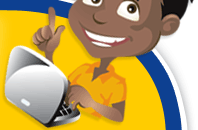# Classroom Key Stage 2 / KS2 - Maths Activities & Resources

## Welcome to the Maths section of the Key Stage 2 classroom. Within KS2 Maths you will find online @school assessment tests that will help children to enhance their learning and revise the subject of Maths at Key Stage 2 level.

•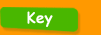•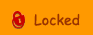•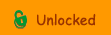••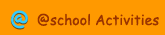•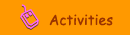•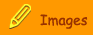###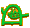Probability

Probability - Interactive activities about Probability from the BBC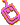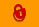Probability - Evens - Interactive activity about the probability of balls.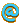Probability - Likelihood - Interactive activity looking at different statements for probability.Probability Clipart - Clipart about probability.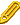Probability Quiz - Interactive revision quiz about ProbabilityRevision Bite  Probability - Online fact sheet about Probability from the BBC